Home / Physics / Converging Lens

# Converging Lens

## Definition: What is a Converging Lens?

A converging lens is an optical lens that converges all rays of light passing through it. The primary purpose of a converging lens is to focus the incoming rays from an object and converge them to form an image. The image can be magnified, diminished, or remain the same depending on the distance of the object from the lens. In many optical systems, converging lenses are used to get a better view of the object.Converging Lens Example

Source: Amazon.com

## Converging Lens Ray Diagram

Terms Used in Converging Lens Ray Diagram

1. Incident ray: The ray of light that is incident on the surface of the lens
2. Refracted ray: The ray of light that is refracted by the lens
3. Center of curvature: The center of the sphere from which the convex lens has been constructed
4. Radius of curvature: The radius of the sphere from which the convex lens has been constructed
5. Pole: The mid-point of the convex lens
6. Principal axis: An imaginary line that connects the pole and the center of curvature
7. Focus: A point on the principal axis where rays of light parallel to the axis converge after refraction
8. Focal length: The distance between the pole and the focus and is one-half of the radius of curvature
9. Object distance: The distance between the object and the pole
10. Image distance: The distance between the image and the pole
11. Magnification: The ratio of the image size to the object size

In order to draw a ray diagram, take two rays of light from the object.

Ray 1: A ray parallel to the principal axis

Ray 2: A ray passing through the pole

After reaching the lens, ray 1 will bend and pass through the focus. Ray 2 will pass without any deviation. The two rays will converge at a point and recreate an image. The position and magnification of the image will depend on the position of the object. A converging lens can produce both real and virtual images.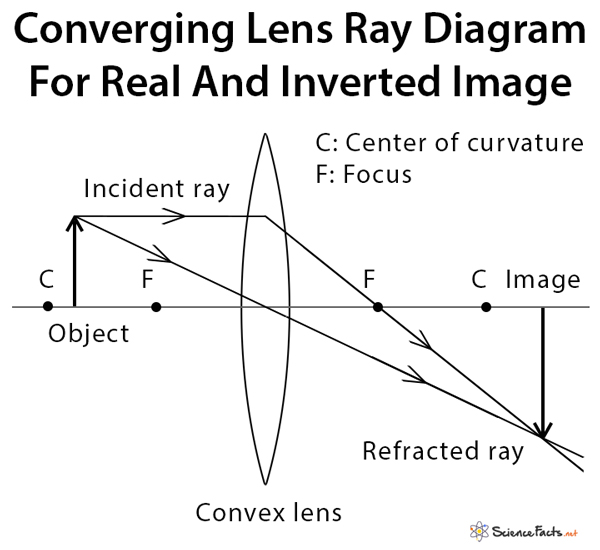Converging Lens Ray Diagram Real Image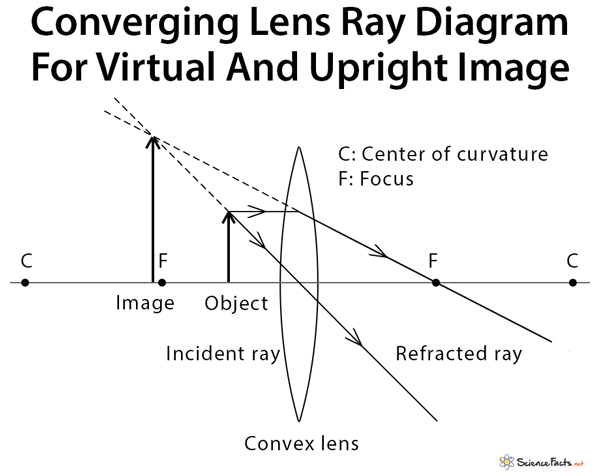Converging Lens Ray Diagram Virtual Image

## Converging Lens Equation

We assume that the lens is thin. Otherwise, a thick lens can cause aberration.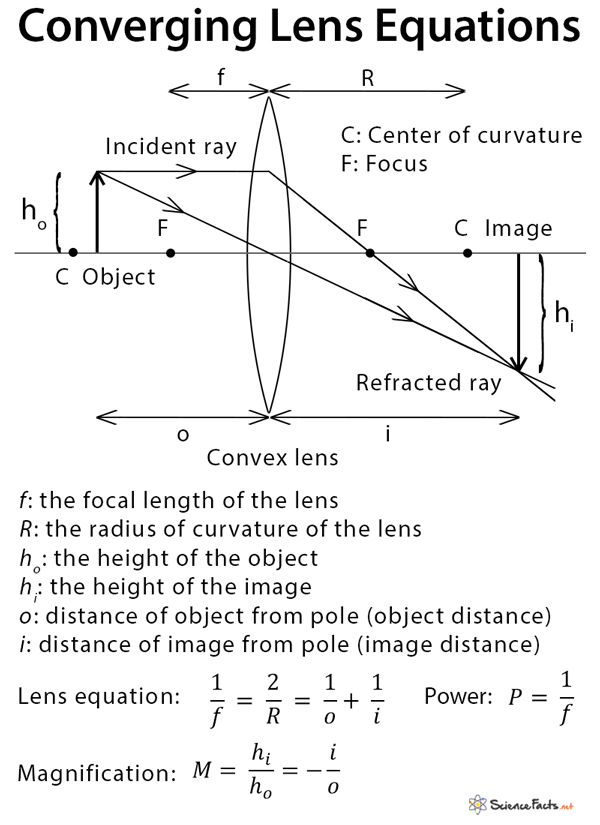Converging Lens Equations

## Applications of Converging Lens

Converging lenses are used in a magnifying glass, eyeglasses (for farsightedness), cameras, and microscope. For example, a magnifying glass produces an image that is upright, magnified, and virtual if the object is at or within the focal length of the lens. Likewise, eyeglasses are used to correct vision by ensuring that the light is focused on the retina instead of behind it.

Article was last reviewed on Tuesday, July 7, 2020

### Related articles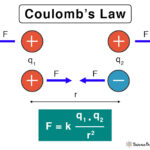Coulomb’s Law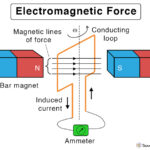Electromagnetic ForceNuclear Force